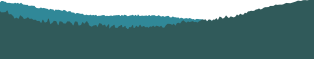PyCon 2016 in Portland, Or## Computational Physics with Python: Planetary Orbits from Newton to Feynman

Audience level:
Novice
Category:
Science

### Description

Newton's explanation of planetary orbits is one of the greatest achievements of science. We will follow Feynman's approach to show how the motion of the planets around the sun can be calculated using computers and without using Newton's advanced mathematics. This talk will convince you that doing physics with Python is way more fun than the way you did physics in high school or university.

### Abstract

Computational Physics has been traditionally done using statically typed compiled languages and efficient but complicated algorithms. This is done to ensure good performance of the code but it increases the barrier for a student. However, with the capabilites of current computers, one can now use Python and straight forward algorithms to learn Computational Physics and to rediscover the great achievements of Physics. In this talk we will follow the computational approach of the Feynman Lectures on Physics in applying Newton's law of gravity to the solar system. This approach allows one to get Newton's results without using the advanced mathematics which was developed by Newton. I will state Newton's laws and show how the laws can be translated to Python code to compute the motion of the planets around the sun. During the talk we will run the code and visualize the orbits of planets using Matplotlib. Note that a similar computational approach is used to compute the trajectories of spacecraft which travel to other planets. Hence it is very exciting that you can actually write your own code to do similar calculations on your own computer. The talk will only use high school math and elementary Python programming skills. The necessary physics will be explained in the talk.